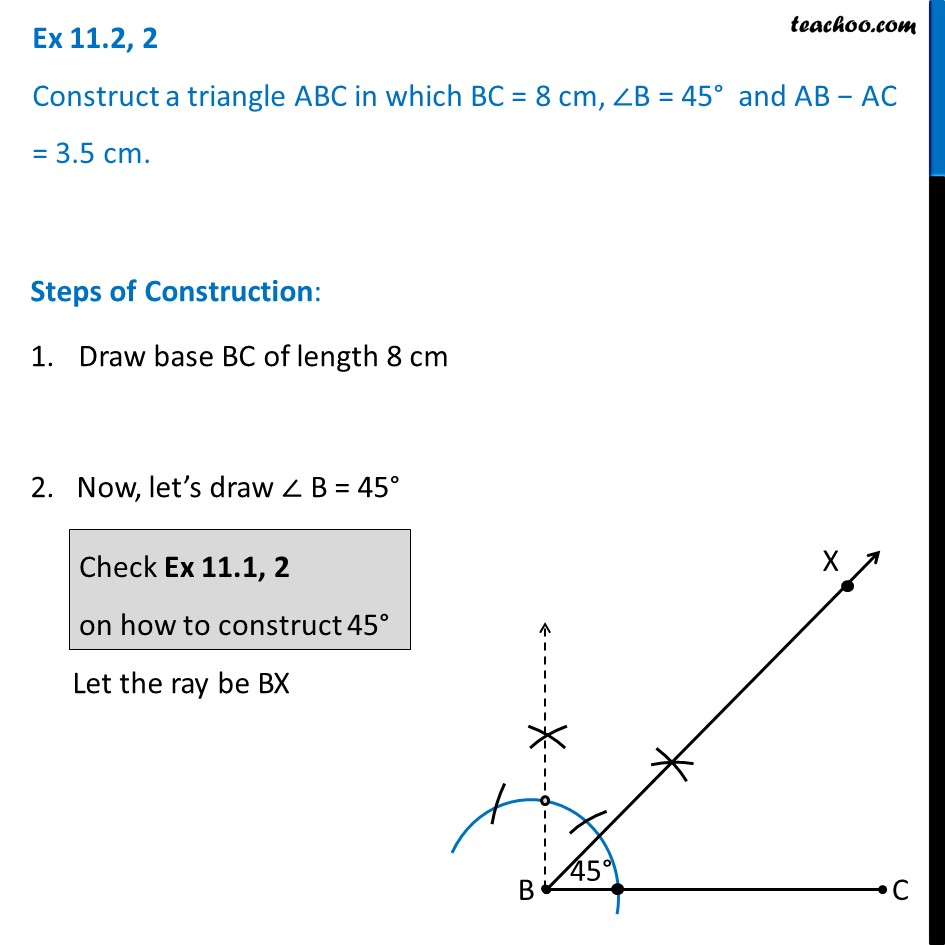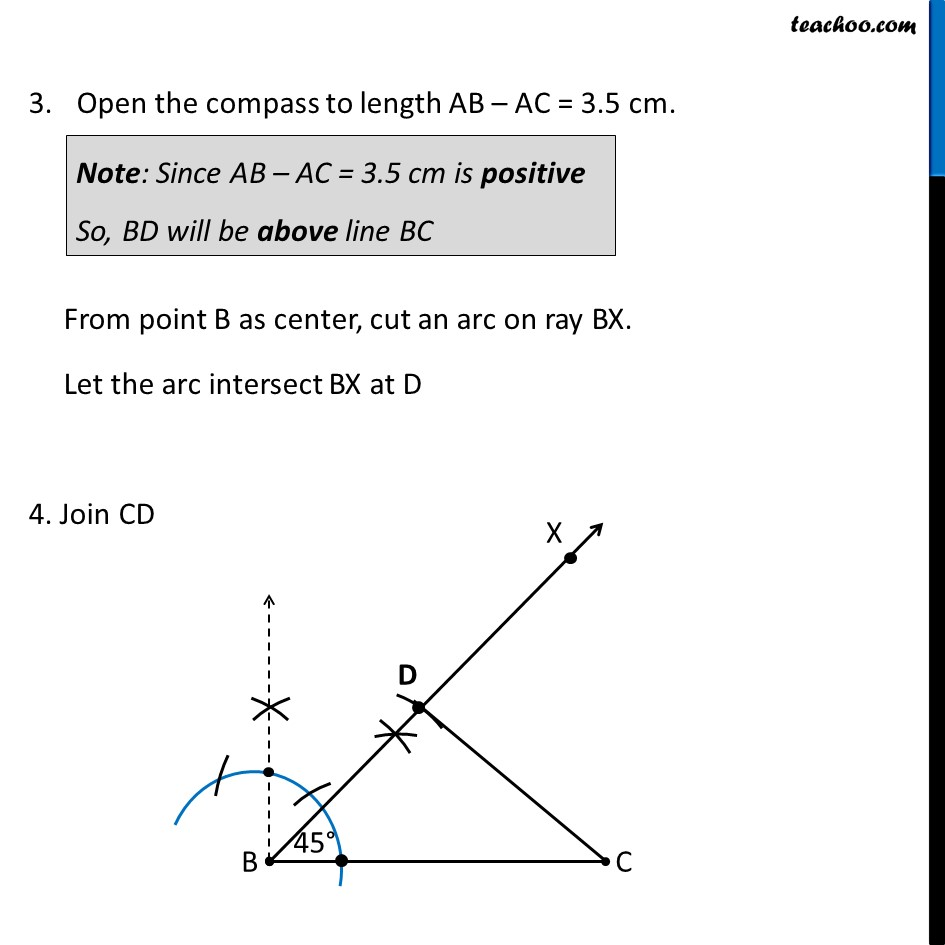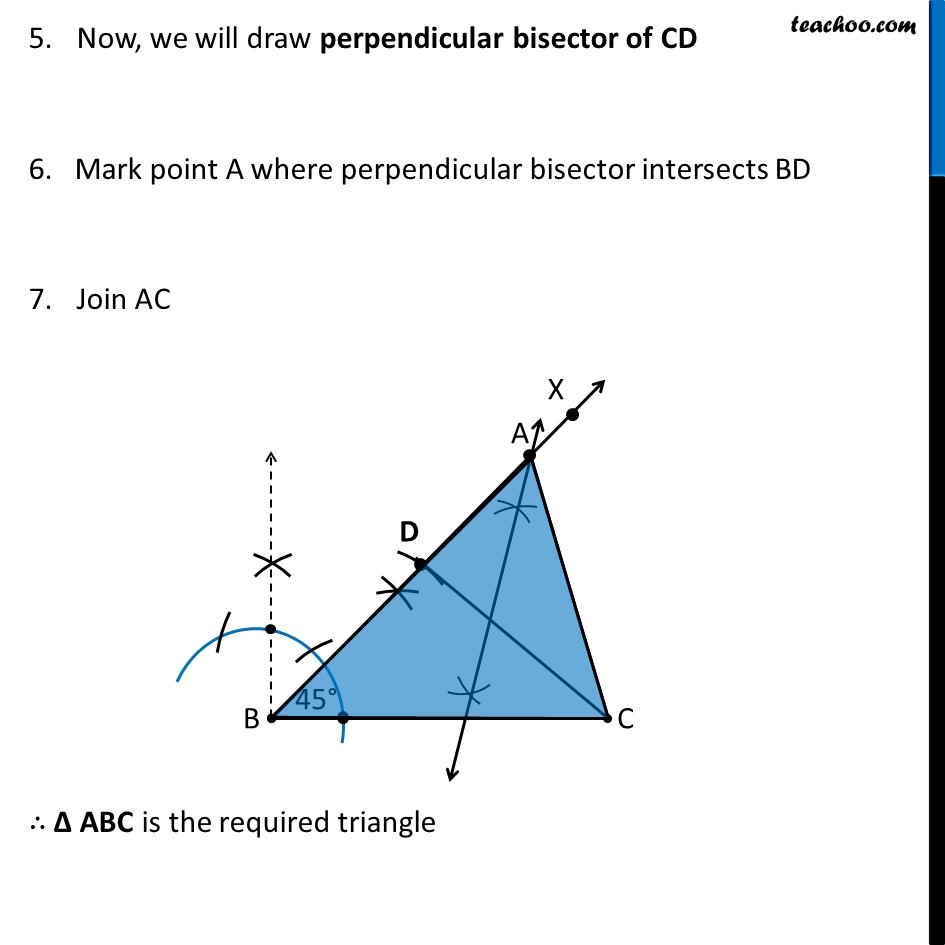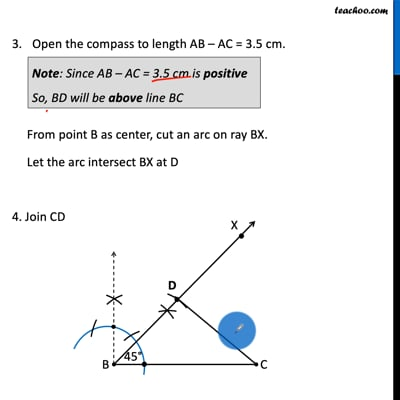Ex 11.2

Chapter 11 Class 9 Constructions [Deleted]
Serial order wiseThis video is only available for Teachoo black users

Maths Crash Course - Live lectures + all videos + Real time Doubt solving!

### Transcript

Ex 11.2, 2 Construct a triangle ABC in which BC = 8 cm, ∠B = 45° and AB − AC = 3.5 cm. Steps of Construction: Draw base BC of length 8 cm 2. Now, let’s draw ∠ B = 45° Let the ray be BX Check Ex 11.1, 2 on how to construct 45° Open the compass to length AB – AC = 3.5 cm. From point B as center, cut an arc on ray BX. Let the arc intersect BX at D 4. Join CD Note: Since AB – AC = 3.5 cm is positive So, BD will be above line BC Now, we will draw perpendicular bisector of CD 6. Mark point A where perpendicular bisector intersects BD Join AC ∴ Δ ABC is the required triangle